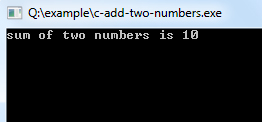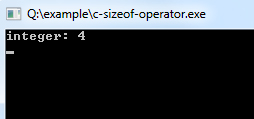## C Programming Tutorial Index

C operators are symbols that are used to perform mathematical or logical manipulations. C programming language is rich with built-in operators. Operators take part in a program for manipulating data and variables and form a part of the mathematical or logical expressions.

## Types of Operators in C

The c programming language offers various types of operators having different functioning capabilities.

### Arithmetic Operators

Arithmetic Operators are used for performing mathematical calculations like addition (`+`), subtraction (`-`), multiplication (`*`), division (`/`), and modulus (`%`).

Operator Description
`+` Addition
`-` Subtraction
`*` Multiplication
`/` Division
`%` Modulus

#### C Program to Add Two Numbers

Example:

``````#include <stdio.h>
void main()
{
int i=3,j=7,k; /* Variables Defining and Assign values */

k=i+j;
printf("sum of two numbers is %d\n", k);
}
``````

Program Output:### Increment and Decrement Operators

Increment and Decrement Operators are useful operators generally used to minimize the calculation, i.e. `++x` and `x++` means `x=x+1` or `-x` and `x--`means `x=x-1`. But there is a slight difference between `++` or `--` written before or after the operand. Applying the pre-increment first adds one to the operand, and then the result is assigned to the variable on the left, whereas post-increment first assigns the value to the variable on the left and then increments the operand.

Operator Description
`++` Increment
`−−` Decrement

#### C Program Demonstrate Prefix and Postfix Modes

``````#include <stdio.h>
//stdio.h is a header file used for input.output purpose.

void main()
{
//set a and b both equal to 5.
int a=5, b=5;

//Print them and decrementing each time.
//Use postfix mode for a and prefix mode for b.
printf("\n%d %d",a--,--b);
printf("\n%d %d",a--,--b);
printf("\n%d %d",a--,--b);
printf("\n%d %d",a--,--b);
printf("\n%d %d",a--,--b);
}``````

Program Output:

```5 4
4 3
3 2
2 1
1 0```

### Relational Operators

Relational operators are used to compare two quantities or values.

Operator Description
`==` Is equal to
`!=` It is not equal to
`>` Greater than
`<` Less than
`>=` Greater than or equal to
`<=` Less than or equal to

### Logical Operators

C provides three logical operators when we test more than one condition to make decisions. These are: `&&` (meaning logical AND), `||` (meaning logical OR) and `!` (meaning logical NOT).

Operator Description
`&&` And operator. It performs logical conjunction of two expressions. (if both expressions evaluate to `True`, the result is `True`. If either expression evaluates to `False`, the result is `False`)
`||` Or operator. It performs a logical disjunction on two expressions. (if either or both expressions evaluate to `True`, the result is `True`)
`!` Not operator. It performs logical negation on an expression.

### Bitwise Operators

C provides a special operator for bit operation between two variables.

Operator Description
`<<` Binary Left Shift Operator
`>>` Binary Right Shift Operator
`~` Binary Ones Complement Operator
`&` Binary AND Operator
`^` Binary XOR Operator
`|` Binary OR Operator

### Assignment Operators

Assignment operators are applied to assign the result of an expression to a variable. C has a collection of shorthand assignment operators.

Operator Description
`=` Assign
`+=` Increments then assign
`-=` Decrements then assign
`*=` Multiplies then assign
`/=` Divides then assign
`%=` Modulus then assign
`<<=` Left shift and assign
`>>=` Right shift and assign
`&=` Bitwise AND assign
`^=` Bitwise exclusive OR and assign
`|=` Bitwise inclusive OR and assign

### Conditional Operator

C offers a ternary operator, which is the conditional operator (`?:` in combination), to construct conditional expressions.

Operator Description
`? :`  Conditional Expression

### Special Operators

C supports some special operators

Operator Description
`sizeof()`  Returns the size of a memory location.
`&`  Returns the address of a memory location.
`*`  Pointer to a variable.

#### Program to Demonstrate the Use of `sizeof()` Operator

Example:

``````#include <stdio.h>
void main()
{
int i=10; // Variables Defining and Assign values
printf("integer: %d\n", sizeof(i));
}
``````

Program Output: